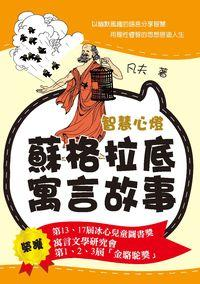### 智慧心燈:蘇格拉底寓言故事

• 點閱：10
• 其他題名：蘇格拉底寓言故事
• 作者：
• 出版年：2012[民101]
• 出版社：要有光出版 秀威資訊科技製作發行 易可數位行銷公司總經銷
• 出版地：臺北市 新北市
• ISBN：978-986-88394-1-0 ; 986-88394-1-6

##簡介

「寓言文學研究會第一、二、三屆『金駱駝獎』」

##章節

• 名家評論(p.5)
• 遠山(p.13)
• 快樂(p.15)
• 人生真諦(p.17)
• 答雞(p.19)
• 蝴蝶的禮物(p.21)
• 起飛的地點(p.23)
• 毛毛蟲迷路(p.24)
• 非學術爭論(p.25)
• 買刀(p.28)
• 蘋果的味兒(p.30)
• 鳥兒收藏家(p.32)
• 廚師家的老鼠(p.34)
• 雪虎(p.36)
• 斑馬的條紋(p.38)
• 心思(p.41)
• 蒙面人(p.43)
• 選擇(p.45)
• 美麗的小鳥(p.47)
• 頭髮(p.49)
• 損失(p.50)
• 狡蚊之死(p.52)
• 讓鳥兒選擇(p.54)
• 丟失(p.56)
• 讚美(p.58)
• 怪題(p.60)
• 尋找名馬(p.63)
• 誰是麗麗(p.65)
• 懸崖上(p.67)
• 百花會(p.69)
• 瘋子(p.71)
• 財富(p.73)
• 公平在心(p.76)
• 球王得子(p.79)
• 眼皮問題(p.81)
• 鼻子(p.83)
• 魚缸(p.85)
• 玉米種子(p.87)
• 抱牛(p.90)
• 讓月亮跟你走(p.92)
• 平息爭鬥(p.94)
• 一碗水(p.96)
• 學會放棄(p.98)
• 角色(p.100)
• 傘(p.102)
• 「美妙」的誘惑(p.104)
• 最簡單的事(p.106)
• 容易丟掉的鑰匙(p.108)
• 心境(p.110)
• 年輕的秘密(p.113)
• 摔跤的地方(p.115)
• 改變(p.117)
• 追求(p.120)
• 不合「群」(p.122)
• 背後的議論(p.124)
• 膽子(p.126)
• 狗性(p.128)
• 名氣(p.130)
• 珍惜(p.132)
• 貧窮的人(p.134)
• 慾望與煩惱(p.137)
• 神藥(p.139)
• 一奧波爾馬車(p.141)
• 一條葉脈(p.143)
• 雅典的小鳥(p.145)
• 瘸蟬(p.147)
• 賽馬(p.150)
• 成功的秘訣(p.152)
• 虎不結幫(p.155)
• 辨識謬誤(p.158)
• 有錢人(p.161)
• 影子(p.163)
• 不湊熱鬧(p.165)
• 落水(p.167)
• 開學第一課(p.169)
• 原因很簡單(p.171)
• 一雙鞋(p.173)
• 這就是原因(p.175)
• 開張的日子(p.178)
• 朋友(p.181)
• 彎絲瓜(p.183)
• 識人(p.185)
• 仇恨者名單(p.187)
• 眼蟲藻的身份(p.190)
• 烈馬(p.193)
• 企鵝村(p.196)
• 一片紙(p.199)
• 路上的「黃金」(p.201)
• 菜中蘭花(p.204)
• 衣著(p.206)
• 不爭論(p.209)
• 生活暢想(p.210)
• 野鴨子定律(p.212)
• 叫太陽發光(p.215)
• 天才作家(p.218)
• 快樂的豬(p.221)
• 遭遇無賴(p.223)
• 人與狗(p.225)
• 從太陽上取火(p.227)
• 神廟上的名言(p.230)
• 感謝莫爾(p.233)
• 另類傷害(p.235)
• 狐狸吃葡萄(p.237)
• 自己的規定(p.239)
• 圈子(p.241)
• 錯誤遮罩儀(p.242)
• 誤導(p.244)
• 男女之間(p.246)
• 應該感謝的人(p.248)
• 一張照片(p.250)
• 家鵝和天鵝(p.252)
• 擇徒(p.254)
• 狗吠(p.256)
• 減價(p.258)
• 跋：感謝您，蘇格拉底(p.259)